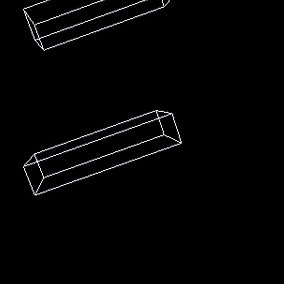# Rigid body game physics 6

A realistic simulation requires the estimation of friction forces.

## Friction Forces

Colliding as well as resting contacts cause constraint impulses as well as friction impulses. So far we have only estimated the constraint impulses of colliding and resting contacts. Constraint impulses are in the direction of the contact's normal vector. Friction impulses act tangential to the contact plane (orthogonal to the normal vector). The inequality constraint isJ and b have two rows. The vectors t1 and t2 are chosen so that they are orthogonal to the normal vector n and orthogonal to each other. Friction forces try to reduce tangential velocities to zero. I.e. b isThe linear components of J are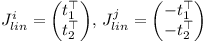and the angular components of J are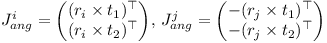As before λ is computed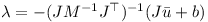Here λ has two elements. λ1 and λ2 are scaled with the same positive factor if necessary so that the length of the 2D vector consisting of λ1 and λ2 is smaller than the normal impulse Pn multiplied with the friction constant μ.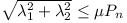Basically the overall force vector needs to reside in the friction cone. In a similar fashion as for constraint impulses, friction impulses are subtracted, recomputed, and then added to the accumulated impulses P of the two objects, when iterating.If you have made it this far, you know how to build a small physics engine!

Any feedback, comments, and suggestions are welcome.